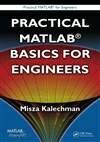# Practical MATLAB Basics for Engineers

A comprehensive and accessible primer, this tutorial immerses engineers and engineering students in the essential technical skills that will allow them to put Matlab® to immediate use. The book covers concepts such as: functions, algebra, geometry, arrays, vectors, matrices, trigonometry, graphs, pre-calculus and calculus. It then delves into the Matlab language, covering syntax rules, notation, operations, computational programming, and general problem solving in the areas of applied mathematics and general physics. This knowledge can be used to explore the basic applications that are detailed in Misza Kalechman’s companion volume, Practical Matlab Applications for Engineers (cat no. 47760). .

# 下载地址

• 本书版权归原作者所有，本站暂无下载，请购买正版！
•